## Info

Publication number
WO2016178270A1
WO2016178270A1 PCT/JP2015/063087 JP2015063087W WO2016178270A1 WO 2016178270 A1 WO2016178270 A1 WO 2016178270A1 JP 2015063087 W JP2015063087 W JP 2015063087W WO 2016178270 A1 WO2016178270 A1 WO 2016178270A1
Authority
WO
WIPO (PCT)
Prior art keywords
doppler
unit
angle
frequency range
processing unit
Prior art date
Application number
PCT/JP2015/063087
Other languages
French (fr)
Japanese (ja)
Inventor

Original Assignee

Priority date (The priority date is an assumption and is not a legal conclusion. Google has not performed a legal analysis and makes no representation as to the accuracy of the date listed.)
Filing date
Publication date
Application filed by 三菱電機株式会社 filed Critical 三菱電機株式会社
Priority to PCT/JP2015/063087 priority Critical patent/WO2016178270A1/en
Publication of WO2016178270A1 publication Critical patent/WO2016178270A1/en

• 238000001228 spectrum Methods 0.000 claims abstract description 35
• 238000000034 methods Methods 0.000 claims abstract description 9
• 230000000694 effects Effects 0.000 description 3

## Images

•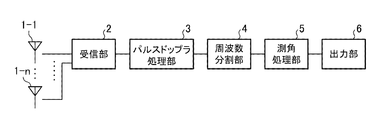•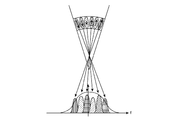••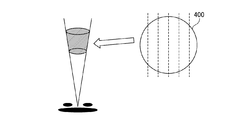•••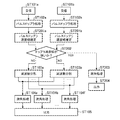•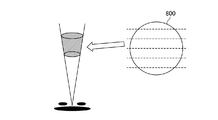•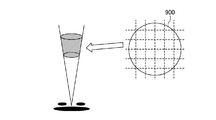## Classifications

• GPHYSICS
• G01MEASURING; TESTING
• G01S13/00Systems using the reflection or reradiation of radio waves, e.g. radar systems; Analogous systems using reflection or reradiation of waves whose nature or wavelength is irrelevant or unspecified
• G01S13/02Systems using reflection of radio waves, e.g. primary radar systems; Analogous systems
• G01S13/06Systems determining position data of a target
• G01S13/42Simultaneous measurement of distance and other co-ordinates
• GPHYSICS
• G01MEASURING; TESTING
• G01S13/00Systems using the reflection or reradiation of radio waves, e.g. radar systems; Analogous systems using reflection or reradiation of waves whose nature or wavelength is irrelevant or unspecified
• G01S13/02Systems using reflection of radio waves, e.g. primary radar systems; Analogous systems
• G01S13/50Systems of measurement based on relative movement of target
• G01S13/52Discriminating between fixed and moving objects or between objects moving at different speeds
• G01S13/522Discriminating between fixed and moving objects or between objects moving at different speeds using transmissions of interrupted pulse modulated waves
• G01S13/524Discriminating between fixed and moving objects or between objects moving at different speeds using transmissions of interrupted pulse modulated waves based upon the phase or frequency shift resulting from movement of objects, with reference to the transmitted signals, e.g. coherent MTI
• GPHYSICS
• G01MEASURING; TESTING
• G01S13/00Systems using the reflection or reradiation of radio waves, e.g. radar systems; Analogous systems using reflection or reradiation of waves whose nature or wavelength is irrelevant or unspecified
• G01S13/95Radar or analogous systems specially adapted for specific applications for meteorological use
• YGENERAL TAGGING OF NEW TECHNOLOGICAL DEVELOPMENTS; GENERAL TAGGING OF CROSS-SECTIONAL TECHNOLOGIES SPANNING OVER SEVERAL SECTIONS OF THE IPC; TECHNICAL SUBJECTS COVERED BY FORMER USPC CROSS-REFERENCE ART COLLECTIONS [XRACs] AND DIGESTS
• Y02TECHNOLOGIES OR APPLICATIONS FOR MITIGATION OR ADAPTATION AGAINST CLIMATE CHANGE
• Y02ATECHNOLOGIES FOR ADAPTATION TO CLIMATE CHANGE
• Y02A90/00Technologies having an indirect contribution to adaptation to climate change
• Y02A90/10Information and communication technologies [ICT] supporting adaptation to climate change.
• Y02A90/12Specially adapted for meteorology, e.g. weather forecasting, climate modelling
• Y02A90/17Weather surveillance systems using the reflection or reradiation of electromagnetic waves

## Abstract

According to the present invention, a frequency dividing unit 4 divides a frequency range of Doppler spectrum output by a pulse Doppler processing unit 3 into a plurality of frequency ranges. An angle measurement processing unit 5 performs an angle measurement process for each of the divided frequency ranges using the Doppler spectrum in the same frequency ranges in the divided frequency ranges. An outputting unit 6 outputs a result of the angle measurement process for each of the divided frequency ranges as a direction for each angle range that is obtained by dividing an angle determined by the beam width of antennas 1-1 to 1-n by the number of divided frequency ranges.

## Description

The present invention relates to a radar apparatus having a resolution exceeding an angular resolution determined by a beam width using an array antenna capable of measuring an angle.

For example, weather radar detects the intensity of echoes reflected by rain particles such as clouds and rain, and uses the Doppler effect of reflected waves to detect dynamic changes in rain and clouds in order to observe or predict weather conditions. A pulse Doppler radar that can be captured is used.

Generally, in pulse Doppler radar, transmission pulses are repeatedly emitted at a required repetition interval. This pulse repetition period is determined by the maximum distance of the target to be detected. In addition, the minimum distance between two targets that can identify different targets in the same direction from the radar as two targets is determined by the transmission pulse width. Furthermore, the minimum azimuth difference between two targets that can identify different targets in the same distance from the radar as two targets is determined by the beam width of the antenna.

Conventionally, weather radar assumes a uniform wind as a premise to blow within the same angle range and the same distance range, and observes the variation in the wind by the Doppler velocity width (for example, non-patent document). 1).

Shoichiro Fukao and Kyosuke Hamatsu, "Radar Remote Sensing of Weather and Atmosphere [Revised 2nd Edition]", Kyoto University Academic Press, July 24, 2009, p. 44-p. 45, p. 108-p. 116.

However, a phenomenon in which the wind direction changes suddenly, such as microburst or wind shear, may have different wind directions within the same angle range and within the same distance range. In this case, the frequency spectrum of the received echo has a total shape in which a plurality of Gaussian distributions are overlapped, so that the Doppler velocity width is widened. When the speed range is wide, it is estimated that the component moves variously, but it is still assumed that the premise is a uniform wind. Therefore, there has been a problem that the difference between the estimation result and the reality becomes large.

In order to deal with such problems, this difference can be reduced by using a higher resolution. However, in order to improve the angle measurement accuracy while obtaining a higher angular resolution, it is necessary to form a narrower beam, which requires an antenna having a sufficient aperture. However, it is not always possible to configure an aerial part having a sufficient opening due to installation location and operational restrictions, and in cases where a beam having a wide shape must be used, sufficient angular resolution can be obtained. There was a problem that it was difficult.

The present invention has been made to solve such a problem, and an object of the present invention is to obtain a radar apparatus capable of realizing sufficient angular resolution even when a beam having a wide shape is used.

A radar apparatus according to the present invention includes a pulse Doppler processing unit that performs pulse Doppler processing on each signal received by a plurality of antennas, and a frequency range of a Doppler spectrum output from the pulse Doppler processing unit. A frequency division unit that divides the frequency range, a doppler spectrum between the same frequency ranges in the divided frequency range, and an angle measurement processing unit that performs angle measurement processing for each divided frequency range, and for each divided frequency range And an output unit that outputs the angle measurement processing result as a direction for each angle range obtained by dividing the angle determined by the beam widths of the plurality of antennas by the number of divisions of the frequency range.

The radar apparatus according to the present invention divides the frequency range of the Doppler spectrum into a plurality of frequency ranges, and performs angle measurement processing for each divided frequency range using the Doppler spectra of the same frequency range in the divided frequency range. It is what I did. Thereby, even when a beam having a wide shape is used, sufficient angular resolution can be realized.

It is a block diagram which shows the radar apparatus of Embodiment 1 of this invention. It is explanatory drawing which shows the concept of the angle measurement process of the radar apparatus of Embodiment 1 of this invention. It is explanatory drawing which shows operation | movement of the radar apparatus of Embodiment 1 of this invention. It is explanatory drawing which shows the division | segmentation of the beam in the radar apparatus of Embodiment 1 of this invention. It is explanatory drawing which shows the relationship between the division | segmentation of the frequency range and the angle measurement process in the radar apparatus of Embodiment 1 of this invention. It is a block diagram which shows the radar apparatus of Embodiment 2 of this invention. It is explanatory drawing which shows operation | movement of the radar apparatus of Embodiment 2 of this invention. It is explanatory drawing which shows the division | segmentation of the beam in the radar apparatus of Embodiment 3 of this invention. It is explanatory drawing which shows the division | segmentation of the beam in the radar apparatus of Embodiment 4 of this invention.

Hereinafter, in order to explain the present invention in more detail, modes for carrying out the present invention will be described with reference to the accompanying drawings.
Embodiment 1 FIG.
FIG. 1 is a configuration diagram of a radar apparatus according to the present embodiment.
As shown in FIG. 1, the radar apparatus according to the present embodiment includes antennas 1-1 to 1-n, a receiving unit 2, a pulse Doppler processing unit 3, a frequency division unit 4, an angle measurement processing unit 5, and an output unit 6. I have. Antennas 1-1 to 1-n are element antennas of an array antenna in which a plurality of element antennas are arranged as transmission antennas or reception antennas of a radar apparatus. In the present embodiment, in order to simplify the description, the following description will be made assuming that the array antenna is composed of two element antennas. The receiving unit 2 is a processing unit that acquires received signals from the antennas 1-1 to 1-n. The configuration of the transmission system in the radar apparatus is not directly related to the present invention, and thus illustration and description thereof are omitted. The pulse Doppler processing unit 3 is a processing unit that performs pulse Doppler processing on the two received signals from the receiving unit 2. The frequency dividing unit 4 divides the Doppler frequency range observable by the radar into a plurality of small ranges and proceeds to the next angle measurement process using only the data of each range, so that the output from the pulse Doppler processing unit 3 A processing unit that divides the signal into a plurality of frequency ranges. In this embodiment, an example of dividing into six parts will be described. The angle measurement processing unit 5 is a processing unit that performs angle measurement processing using signals in the same frequency range divided by the frequency division unit 4. The output unit 6 determines the direction for each angle range obtained by dividing the angle determined by the beam width of the antennas 1-1 to 1-n by the number of divisions in the frequency division unit 4 based on the angle measurement processing result in the angle measurement processing unit 5. As a processing unit that outputs as

FIG. 2 is an explanatory diagram showing the concept of angle measurement processing of the radar apparatus according to the first embodiment. In the first embodiment, the two antenna elements are divided into equal frequency ranges, and angle measurement processing is performed using the data of each range, thereby associating the spectrum and direction of each Gaussian distribution. it can. That is, it is possible to correlate from which part within the same distance range the wind having each divided Doppler frequency is.

Next, the operation of the radar apparatus according to the first embodiment will be described.
FIG. 3 is an explanatory diagram illustrating the operation of the radar apparatus according to the first embodiment.
Radio waves are transmitted from the two antennas 1-1 to 1-2, and reflected waves from the target are received by the receiving unit 2 (steps ST101a and ST101b), and each signal is subjected to pulse Doppler processing by the pulse Doppler processing unit 3. (Steps ST102a and ST102b). Here, the pulse Doppler process is a process for obtaining the Doppler spectrum from the signal received by the receiving unit 2, and since this is known, a detailed description thereof is omitted here. Next, the frequency dividing unit 4 divides the Doppler spectrum output from the pulse Doppler processing unit 3 into six frequency ranges, respectively (Steps ST103a and ST103b). Thereby, the spectrum of each Gaussian distribution is reproduced from the total spectrum in which a plurality of Gaussian distributions overlap. As shown in FIG. 4, this corresponds to dividing the beam 400 viewed from the front into six equal parts in the horizontal direction as shown by broken lines, that is, azimuthally divided into six parts. FIG. 5 is an explanatory diagram of frequency range division in the frequency division unit 4. As shown in FIG. 5, the Doppler spectrum from the frequency division unit 4 is divided into six frequency ranges, and two data in the same frequency range are output to the angle measurement processing unit 5.

The angle measurement processing unit 5 performs angle measurement processing using Doppler spectra in the same frequency range of the six Doppler spectra output from the frequency division unit 4 (steps ST104a to ST104f). That is, the angle measurement processing unit 5 estimates the arrival direction of the incoming wave from which direction the signal is within the range of each of the six beam widths divided by the arrival direction estimation method. Here, in the arrival direction estimation method, the output of each element antenna is multiplied by an appropriate complex weight. That is, by appropriately manipulating the amplitude and phase of the received signal of each element antenna, the directivity of the array antenna can be given to an arbitrary directivity. In this way, the received signal strength with each azimuth within the detection range as the directional azimuth is detected, and the directional azimuth at which the received signal strength peaks among the directional azimuths is estimated as the arrival azimuth of the incoming wave. Further, the arrival direction of the incoming wave may be estimated with the NULL point of the directivity characteristic directed to an arbitrary direction. Various methods such as Beamformer method, Capon method and MUSIC (Multiple Signal Classification) method are known as arrival direction estimation methods. These arrival direction estimation methods are selected according to conditions and the like. By such angle measurement processing, the spectrum and direction of each Gaussian distribution can be associated.

The output unit 6 associates the result of each angle measurement processing divided into 6 with which part of the divided Doppler frequency wind is within the same angle range and the same distance range. Output (step ST105).

As described above, according to the radar apparatus of the first embodiment, a pulse Doppler processing unit that performs pulse Doppler processing on each signal received by a plurality of antennas, and a Doppler output from the pulse Doppler processing unit. Angle measurement processing that performs angle measurement processing for each divided frequency range using a frequency division unit that divides the frequency range of the spectrum into a plurality of frequency ranges, and a Doppler spectrum between the same frequency ranges in the divided frequency ranges And an output unit for outputting the angle measurement processing results for each divided frequency range as directions for each angle range obtained by dividing the angle determined by the beam widths of the plurality of antennas by the number of divided frequency ranges. In the same angle range and the same distance range, it can be further divided into multiple areas, which are determined by the beam width It is possible to realize a resolution exceeding the time resolution, for example, it is possible to realize a sufficient resolution even when performing estimation of sudden weather change such as a microburst or wind shear.

Further, according to the radar apparatus of the first embodiment, since the direction for each angle range obtained by dividing the angle determined by the beam widths of the plurality of antennas by the number of divisions of the frequency range is the horizontal direction, Within the same distance range, it is possible to realize a resolution exceeding the resolution of the direction determined by the beam width. By improving the azimuth resolution, for example, the effect can be expected particularly when the wind direction changes in a layered manner with respect to the azimuth.

Embodiment 2. FIG.
In the first embodiment, the method is effective in a state where the spectrum width is wide. However, when the spectrum width is narrow, there is a high possibility that the wind is uniform in the same angle range and the same distance range. In that case, it may not be effective to perform the processing of the first embodiment. Therefore, in the second embodiment, when the received signal obtained for each element antenna is subjected to pulse Doppler processing, if the Doppler velocity width is narrow, angle measurement processing is performed without dividing the range into a plurality of frequencies. It is.

FIG. 6 is a block diagram showing the configuration of the radar apparatus according to Embodiment 2 of the present invention. The radar apparatus according to the second embodiment includes antennas 1-1 to 1-n, a receiving unit 2, a pulse Doppler processing unit 3, a frequency dividing unit 4, an angle measurement processing unit 5, an output unit 6, a Doppler velocity width estimation unit 7, A Doppler speed width comparison unit 8 is provided. The Doppler velocity width estimation unit 7 is a processing unit that estimates the frequency range of the Doppler spectrum processed by the pulse Doppler processing unit 3, that is, the Doppler velocity width, and the Doppler velocity width comparison unit 8 estimates the Doppler velocity width estimation unit 7. It is determined whether or not the Doppler velocity width is narrower than the set value. If it is narrow, the Doppler spectrum in the pulse Doppler processing unit 3 is output to the angle measurement processing unit 5 as it is, and if it is greater than or equal to the set value , A processing unit that outputs to the frequency division unit 4. In addition, about a setting value, it selects suitably. Further, in FIG. 6, since each other configuration is the same as the configuration of the first embodiment shown in FIG. 1, the same reference numerals are given to the corresponding portions, and the description thereof is omitted.

Next, the operation of the radar apparatus according to the second embodiment will be described.
FIG. 7 is an explanatory diagram showing the operation of the radar apparatus according to the second embodiment.
First, the reception operation (steps ST101a and ST101b) and the pulse Doppler process (steps ST102a and ST102b) are the same as those in the first embodiment. Next, in the second embodiment, the Doppler velocity width estimation unit 7 estimates the frequency width (Doppler velocity width) of the Doppler spectrum output from the pulse Doppler processing unit 3 (Steps ST201a and ST201b), and compares the Doppler velocity widths. The unit 8 determines whether or not the frequency width is narrower than the set value (step ST202). In step ST202, if the frequency width is equal to or larger than the set value, the same operation as in the first embodiment is performed (steps ST103a, ST103b, steps ST104a to ST104f, step ST105).

On the other hand, if the frequency width of the Doppler spectrum is narrower than the set value in step ST202, the frequency division unit 4 does not perform frequency division, and the angle measurement processing unit 5 outputs the Doppler spectrum signal output from the pulse Doppler processing unit 3. Is used for angle measurement processing (step ST203). Next, the output unit 6 outputs the result assuming that the azimuth obtained in step ST203 is the wind from any part within the angle determined by the beam width of the antennas 1-1 to 1-n (step ST204). ).

As described above, according to the radar apparatus of the second embodiment, the pulse Doppler processing unit that performs pulse Doppler processing on each signal received by a plurality of antennas, and the Doppler output from the pulse Doppler processing unit. The Doppler velocity width estimation unit for estimating the spectrum frequency range, the Doppler velocity width comparison unit for determining whether the frequency range estimated by the Doppler velocity width estimation unit is narrower than the set value, and the determination results of the Doppler velocity width comparison unit are If it is greater than or equal to the set value, the determination result of the frequency division unit that divides the frequency range of the Doppler spectrum output from the pulse Doppler processing unit into a plurality of frequency ranges and the Doppler velocity width comparison unit is greater than or equal to the set value. If so, the frequency divided using the Doppler spectrum of the same frequency range in the divided frequency range Angle measurement processing is performed for each range, and if the determination result of the Doppler velocity width comparison unit is narrower than the set value, the angle measurement processing unit performs angle measurement processing using the Doppler spectrum output from the pulse Doppler processing unit, and the division Output the angle measurement processing results for each frequency range as the direction for each angle range obtained by dividing the angle determined by the beam widths of the plurality of antennas by the number of divisions of the frequency range. The resolution exceeding the angular resolution determined by the beam width can be realized while reducing the calculation load within and within the same distance range.

Embodiment 3 FIG.
In the first embodiment and the second embodiment, it is assumed that the angle is measured with respect to the horizontal direction of the received beam. However, the vertical direction is the same as in the first or second embodiment. Can be measured, and this will be described as a third embodiment.

In the estimation from the horizontal direction, the beam is divided into a vertically long range, but in the estimation from the vertical direction, the beam is divided into a horizontally long range. That is, when the angle is measured in the vertical direction, the azimuth resolution is not different from the conventional one, but the resolution of the elevation angle is improved. FIG. 8 shows the concept of beam splitting corresponding to the frequency splitting unit 4 when splitting in the vertical direction. As shown in the figure, the beam 800 when viewed from the front is divided into six equal parts in the vertical direction as indicated by broken lines. The configuration of the radar apparatus is the same as that shown in the first embodiment or the second embodiment, except that angle measurement is performed in the vertical direction.

As described above, according to the radar apparatus of the third embodiment, the direction determined for each angle range obtained by dividing the angle determined by the beam widths of the plurality of antennas by the number of divided frequency ranges is the vertical direction. A resolution exceeding the resolution of the elevation angle determined by the beam width can be realized within the angle range and within the same distance range. By improving the resolution of the elevation angle, for example, an effect can be expected particularly when the wind direction changes in layers with respect to altitude.

Embodiment 4 FIG.
In the first to third embodiments, the direction of angle measurement is performed in either the horizontal direction or the vertical direction. However, when the radar apparatus has a function capable of measuring the angle in two dimensions, the direction of the angle is determined in both directions. On the other hand, angle measurement processing may be performed, which will be described below as a fourth embodiment.

FIG. 9 shows the concept of beam splitting corresponding to the frequency splitting unit 4 when splitting in both the horizontal and vertical directions. As shown in the figure, the beam 900 when viewed from the front is divided into six equal parts in the horizontal direction and the vertical direction as indicated by broken lines. The configuration of the radar apparatus is the same as that shown in the first embodiment or the second embodiment, except that angle measurement is performed in each of the horizontal direction and the vertical direction. With this configuration, the azimuth resolution is improved by the angle measurement in the horizontal direction, and the resolution of the elevation angle is improved by the angle measurement in the vertical direction.

As described above, according to the radar apparatus of the fourth embodiment, the direction for each angle range obtained by dividing the angle determined by the beam widths of the plurality of antennas by the number of divided frequency ranges is defined as the horizontal direction and the vertical direction. Therefore, when angle measurement processing is performed in both the horizontal direction and the vertical direction, a finer grid can be obtained within the same angle range and the same distance range by matching the wind direction and direction output for each direction. State output is possible.

In the present invention, within the scope of the invention, free combinations of the respective embodiments, modifications of arbitrary components of the respective embodiments, or omission of arbitrary components of the respective embodiments are possible. .

As described above, the radar apparatus according to the present invention relates to a radar apparatus having a resolution exceeding the angular resolution determined by the beam width, and is useful for estimating a sudden weather change such as a microburst or wind shear. It is.

1-1 to 1-n antenna, 2 receiving unit, 3 pulse Doppler processing unit, 4 frequency division unit, 5 angle measurement processing unit, 6 output unit, 7 Doppler velocity width estimation unit, 8 Doppler velocity width comparison unit.

## Claims (4)

1. A pulse Doppler processing unit that performs pulse Doppler processing on each signal received by a plurality of antennas;
A frequency division unit that divides the frequency range of the Doppler spectrum output from the pulse Doppler processing unit into a plurality of frequency ranges;
Using a Doppler spectrum between the same frequency ranges in the divided frequency range, an angle measurement processing unit that performs angle measurement processing for each of the divided frequency ranges;
A radar having an output unit that outputs the angle measurement processing result for each divided frequency range as a direction for each angle range obtained by dividing an angle determined by a beam width of the plurality of antennas by a number of divisions of the frequency range; apparatus.
2. A pulse Doppler processing unit that performs pulse Doppler processing on each signal received by a plurality of antennas;
A Doppler velocity width estimation unit for estimating a frequency range of a Doppler spectrum output from the pulse Doppler processing unit;
A Doppler speed width comparison unit for determining whether the frequency range estimated by the Doppler speed width estimation unit is narrower than a set value;
When the determination result of the Doppler velocity width comparison unit is a set value or more, a frequency division unit that divides the frequency range of the Doppler spectrum output from the pulse Doppler processing unit into a plurality of frequency ranges,
When the determination result of the Doppler speed width comparison unit is equal to or greater than a set value, the angle measurement process is performed for each divided frequency range using a Doppler spectrum between the same frequency ranges in the divided frequency range, When the determination result of the Doppler velocity width comparison unit is narrower than a set value, an angle measurement processing unit that performs angle measurement processing using the Doppler spectrum output from the pulse Doppler processing unit,
A radar having an output unit that outputs the angle measurement processing result for each divided frequency range as a direction for each angle range obtained by dividing an angle determined by a beam width of the plurality of antennas by a number of divisions of the frequency range; apparatus.
3. The radar apparatus according to claim 1, wherein the direction for each angle range obtained by dividing the angle determined by the beam widths of the plurality of antennas by the number of divisions of the frequency range is a horizontal direction or a vertical direction.
4. The radar apparatus according to claim 1, wherein the direction for each angle range obtained by dividing the angle determined by the beam width of the plurality of antennas by the number of divisions of the frequency range is a horizontal direction and a vertical direction.
PCT/JP2015/063087 2015-05-01 2015-05-01 Radar device WO2016178270A1 (en)

## Priority Applications (1)

Application Number Priority Date Filing Date Title
PCT/JP2015/063087 WO2016178270A1 (en) 2015-05-01 2015-05-01 Radar device

## Applications Claiming Priority (1)

Application Number Priority Date Filing Date Title
PCT/JP2015/063087 WO2016178270A1 (en) 2015-05-01 2015-05-01 Radar device

## Publications (1)

Publication Number Publication Date
WO2016178270A1 true WO2016178270A1 (en) 2016-11-10

# Family

## Family Applications (1)

Application Number Title Priority Date Filing Date
PCT/JP2015/063087 WO2016178270A1 (en) 2015-05-01 2015-05-01 Radar device

## Country Status (1)

WO (1) WO2016178270A1 (en)

## Citations (4)

* Cited by examiner, † Cited by third party
Publication number Priority date Publication date Assignee Title
JP2007147532A (en) * 2005-11-30 2007-06-14 Hitachi Ltd Radar system
JP2009156807A (en) * 2007-12-27 2009-07-16 Toshiba Corp Angle measuring device
JP2009300207A (en) * 2008-06-12 2009-12-24 Nagano Japan Radio Co Ocean radar station and device for ocean radar observation
JP2010256333A (en) * 2009-04-02 2010-11-11 Toshiba Corp Weather radar and weather observation method

## Patent Citations (4)

* Cited by examiner, † Cited by third party
Publication number Priority date Publication date Assignee Title
JP2007147532A (en) * 2005-11-30 2007-06-14 Hitachi Ltd Radar system
JP2009156807A (en) * 2007-12-27 2009-07-16 Toshiba Corp Angle measuring device
JP2009300207A (en) * 2008-06-12 2009-12-24 Nagano Japan Radio Co Ocean radar station and device for ocean radar observation
JP2010256333A (en) * 2009-04-02 2010-11-11 Toshiba Corp Weather radar and weather observation method

## Similar Documents

Publication Publication Date Title
US9562968B2 (en) Sensor system and method for determining target location using sparsity-based processing
US6081220A (en) Radar system for observing weather phenomena with improved radar system parameters
JP4737165B2 (en) Radar target detection method and radar apparatus using the target detection method
Malanowski et al. Parade-passive radar demonstrator family development at warsaw university of technology
US20160069994A1 (en) Sense-and-avoid systems and methods for unmanned aerial vehicles
JP6192910B2 (en) Radar apparatus and altitude calculation method
US7796082B2 (en) Methods and apparatus for log-FTC radar receivers having enhanced sea clutter model
Yu et al. Beam multiplexing using the phased-array weather radar
RU2440588C1 (en) Passive radio monitoring method of air objects
EP2491418B1 (en) Resolution enhancement system for networked radars
Malanowski et al. Analysis of detection range of FM-based passive radar
EP2087368B1 (en) Method and system for detecting signal sources in a surveillance space
US4996533A (en) Single station radar ocean surface current mapper
US20170222735A1 (en) Massive-mimo antenna measurement device and method of measuring directivity thereof
US20100245157A1 (en) Generalized inner product method and apparatus for improved detection and discrimination
US8970426B1 (en) Automatic matched Doppler filter selection
US8884810B2 (en) Compact beacon radar and full ATC services system
US9329266B2 (en) Weather radar apparatus, observation sequence generation method, and observation sequence generation program
US7961147B1 (en) Long baseline phase interferometer ambiguity resolution using frequency differences
JP6037762B2 (en) Wind measuring device

## Legal Events

Date Code Title Description
121 Ep: the epo has been informed by wipo that ep was designated in this application

Ref document number: 15891277

Country of ref document: EP

Kind code of ref document: A1

NENP Non-entry into the national phase in:

Ref country code: DE

122 Ep: pct application non-entry in european phase

Ref document number: 15891277

Country of ref document: EP

Kind code of ref document: A1

NENP Non-entry into the national phase in:

Ref country code: JP